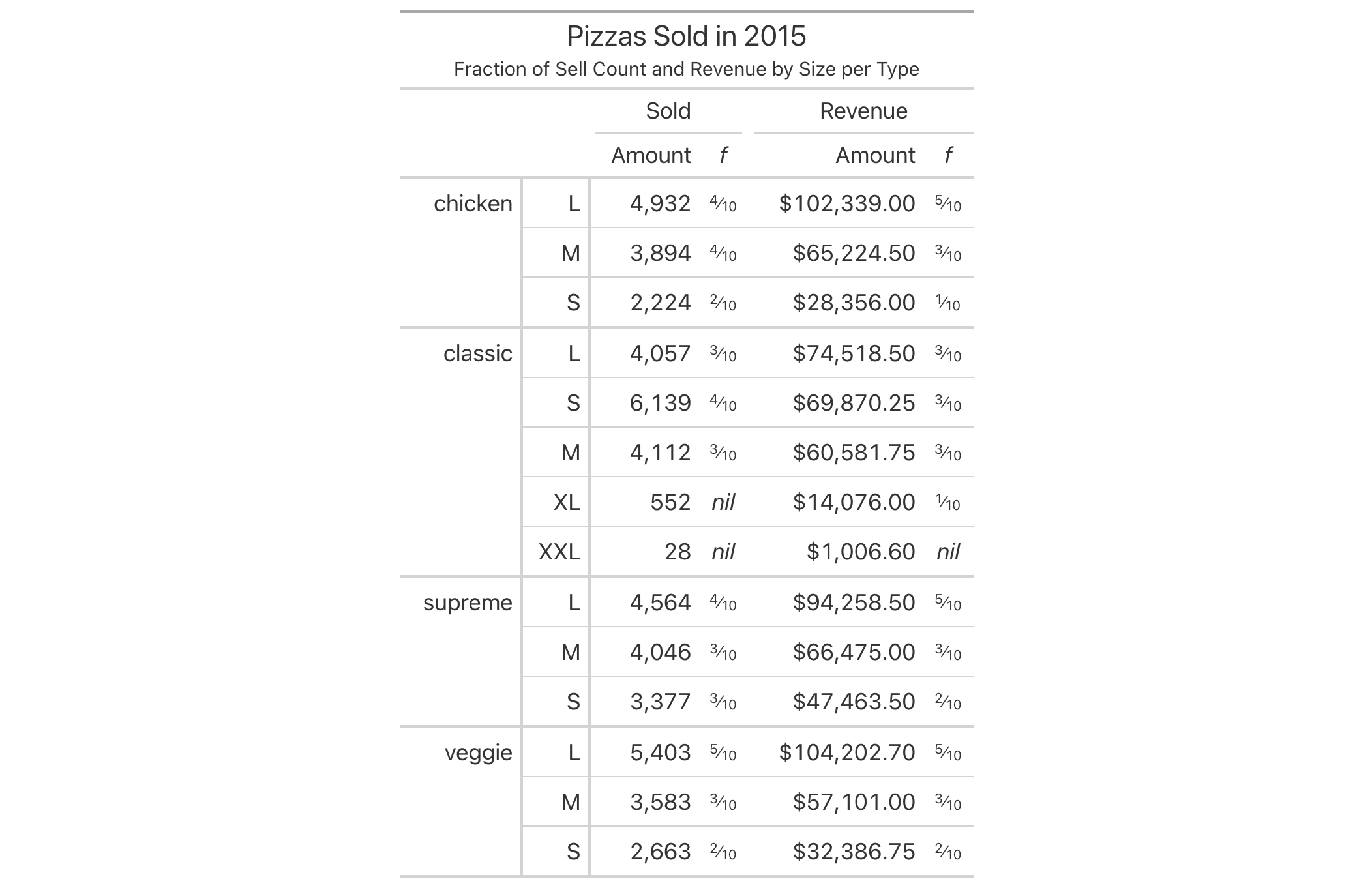With numeric values in a gt table, we can perform mixed-fraction-based formatting. There are several options for setting the accuracy of the fractions. Furthermore, there is an option for choosing a layout (i.e., typesetting style) for the mixed-fraction output.

The following options are available for controlling this type of formatting:

• accuracy: how to express the fractional part of the mixed fractions; there are three keyword options for this and an allowance for arbitrary denominator settings

• simplification: an option to simplify fractions whenever possible

• layout: We can choose to output values with diagonal or inline fractions

• digit grouping separators: options to enable/disable digit separators and provide a choice of separator symbol for the whole number portion

• pattern: option to use a text pattern for decoration of the formatted mixed fractions

• locale-based formatting: providing a locale ID will result in number formatting specific to the chosen locale

## Usage

fmt_fraction(
data,
columns,
rows = everything(),
accuracy = NULL,
simplify = TRUE,
layout = c("inline", "diagonal"),
use_seps = TRUE,
pattern = "{x}",
sep_mark = ",",
system = c("intl", "ind"),
locale = NULL
)

## Arguments

data

A table object that is created using the gt() function.

columns

The columns to format. Can either be a series of column names provided in c(), a vector of column indices, or a helper function focused on selections. The select helper functions are: starts_with(), ends_with(), contains(), matches(), one_of(), num_range(), and everything().

rows

Optional rows to format. Providing everything() (the default) results in all rows in columns being formatted. Alternatively, we can supply a vector of row captions within c(), a vector of row indices, or a helper function focused on selections. The select helper functions are: starts_with(), ends_with(), contains(), matches(), one_of(), num_range(), and everything(). We can also use expressions to filter down to the rows we need (e.g., [colname_1] > 100 & [colname_2] < 50).

accuracy

The type of fractions to generate. This can either be one of the keywords "low", "med", or "high" (to generate fractions with denominators of up to 1, 2, or 3 digits, respectively) or an integer value greater than zero to obtain fractions with a fixed denominator (2 yields halves, 3 is for thirds, 4 is quarters, etc.). For the latter option, using simplify = TRUE will simplify fractions where possible (e.g., 2/4 will be simplified as 1/2). By default, the "low" option is used.

simplify

If choosing to provide a numeric value for accuracy, the option to simplify the fraction (where possible) can be taken with TRUE (the default). With FALSE, denominators in fractions will be fixed to the value provided in accuracy.

layout

For HTML output, the "inline" layout is the default. This layout places the numerals of the fraction on the baseline and uses a standard slash character. The "diagonal" layout will generate fractions that are typeset with raised/lowered numerals and a virgule.

use_seps

An option to use digit group separators. The type of digit group separator is set by sep_mark and overridden if a locale ID is provided to locale. This setting is TRUE by default.

pattern

A formatting pattern that allows for decoration of the formatted value. The value itself is represented by {x} and all other characters are taken to be string literals.

sep_mark

The mark to use as a separator between groups of digits (e.g., using sep_mark = "," with 1000 would result in a formatted value of 1,000).

system

The numbering system to use. By default, this is the international numbering system ("intl") whereby grouping separators (i.e., sep_mark) are separated by three digits. The alternative system, the Indian numbering system ("ind") uses grouping separators that correspond to thousand, lakh, crore, and higher quantities.

locale

An optional locale ID that can be used for formatting the value according the locale's rules. Examples include "en" for English (United States) and "fr" for French (France). The use of a valid locale ID will override any values provided in sep_mark and dec_mark. We can use the info_locales() function as a useful reference for all of the locales that are supported. Any locale value provided here will override any global locale setting performed in gt()'s own locale argument.

## Value

An object of class gt_tbl.

## Targeting the values to be formatted

Targeting of values is done through columns and additionally by rows (if nothing is provided for rows then entire columns are selected). Conditional formatting is possible by providing a conditional expression to the rows argument. See the Arguments section for more information on this.

## Examples

Use pizzaplace to create a gt table. Format the f_sold and f_income columns to display fractions.

pizzaplace %>%
dplyr::group_by(type, size) %>%
dplyr::summarize(
sold = dplyr::n(),
income = sum(price),
.groups = "drop_last"
) %>%
dplyr::group_by(type) %>%
dplyr::mutate(
f_sold = sold / sum(sold),
f_income = income / sum(income),
) %>%
dplyr::arrange(type, dplyr::desc(income)) %>%
gt(rowname_col = "size") %>%
title = "Pizzas Sold in 2015",
subtitle = "Fraction of Sell Count and Revenue by Size per Type"
) %>%
fmt_integer(columns = sold) %>%
fmt_currency(columns = income) %>%
fmt_fraction(
columns = starts_with("f_"),
accuracy = 10,
simplify = FALSE,
layout = "diagonal"
) %>%
sub_missing(missing_text = "") %>%
tab_spanner(
label = "Sold",
columns = contains("sold")
) %>%
tab_spanner(
label = "Revenue",
columns = contains("income")
) %>%
text_transform(
locations = cells_body(),
fn = function(x) {
dplyr::case_when(
x == 0 ~ "<em>nil</em>",
x != 0 ~ x
)
}
) %>%
cols_label(
sold = "Amount",
income = "Amount",
f_sold = md("_f_"),
f_income = md("_f_")
) %>%
cols_align(align = "center", columns = starts_with("f")) %>%
tab_options(
table.width = px(400),
row_group.as_column = TRUE
)## Function ID

3-7

Other data formatting functions: data_color(), fmt_bytes(), fmt_currency(), fmt_datetime(), fmt_date(), fmt_duration(), fmt_engineering(), fmt_integer(), fmt_markdown(), fmt_number(), fmt_partsper(), fmt_passthrough(), fmt_percent(), fmt_roman(), fmt_scientific(), fmt_time(), fmt(), sub_large_vals(), sub_missing(), sub_small_vals(), sub_values(), sub_zero(), text_transform()